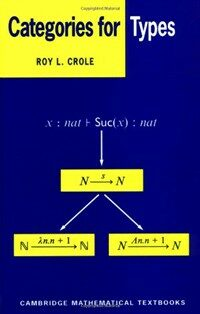> 상세정보

상세정보Categories for types (1회 대출)

자료유형
단행본
개인저자
Crole, Roy L.
서명 / 저자사항
Categories for types / Roy L. Crole.
발행사항
Cambridge ;   New York :   Cambridge University Press ,   1993   (2002 printing)
형태사항
xvii, 335 p. : ill. ; 24 cm.
총서사항
Cambridge mathematical textbooks
ISBN
0521450926 0521457017 (pbk.) 9780521457019 (pbk.)
서지주기
Includes bibliographical references (p. 315-319) and index.
일반주제명
Categories (Mathematics) Lambda calculus.
 000 00972camuu2200301 a 4500 001 000045453827 005 20080717105440 008 940718s1993 enka b 001 0 eng 010 ▼a 94184387 020 ▼a 0521450926 020 ▼a 0521457017 (pbk.) 020 ▼a 9780521457019 (pbk.) 035 ▼a (KERIS)REF000006662286 040 ▼a DLC ▼c DLC ▼d DLC ▼d 211009 050 0 0 ▼a QA169 ▼b .C685 1993 082 0 0 ▼a 511.3 ▼2 20 082 0 4 ▼a 512.62 ▼2 22 090 ▼a 512.62 ▼b C944c 100 1 ▼a Crole, Roy L. 245 1 0 ▼a Categories for types / ▼c Roy L. Crole. 260 ▼a Cambridge ; ▼a New York : ▼b Cambridge University Press , ▼c 1993 ▼g (2002 printing) 300 ▼a xvii, 335 p. : ▼b ill. ; ▼c 24 cm. 440 0 ▼a Cambridge mathematical textbooks 504 ▼a Includes bibliographical references (p. 315-319) and index. 650 0 ▼a Categories (Mathematics) 650 0 ▼a Lambda calculus. 945 ▼a KINS

소장정보

No. 소장처 청구기호 등록번호 도서상태 반납예정일 예약 서비스
No. 1 소장처 청구기호 512.62 C944c 등록번호 121173247 도서상태 대출가능 반납예정일 예약 서비스

컨텐츠정보

목차

CONTENTS
Preface = ⅹ
1 Order, Lattices and Domains = 1
1.1 Introduction = 1
1.2 Ordered Sets = 2
1.3 Basic Lattice Theory = 12
1.4 Boolean and Heyting Lattices = 20
1.5 Elementary Domain Theory = 24
1.6 Further Exercises = 33
1.7 Pointers to the Literature = 36
2 A Category Theory Primer = 37
2.1 Introduction = 37
2.2 Categories and Examples = 40
2.3 Functors and Examples = 45
2.4 Natural Transformations and Examples = 49
2.5 Isomorphisms and Equivalences = 52
2.6 Products and Coproducts = 55
2.7 The Yoneda Lemma = 61
2.8 Cartesian Closed Categories = 67
2.9 Monics, Equalisers, Pullbacks and their Duals = 73
2.11 Limits and Colimits = 91
2.12 Strict Indexed Categories = 106
2.13 Further Exercises = 113
2.14 Pointers to the Literature = 118
3 Algebraic Type Theory = 120
3.1 Introduction = 120
3.2 Definition of the Syntax = 121
3.3 Algebraic Theories = 127
3.4 Motivating a Categorical Semantics = 129
3.5 Categorical Semantics = 132
3.6 Categorical Models and the Soundness Theorem = 134
3.7 Categories of Models = 137
3.8 Classifying Category of an Algebraic Theory = 139
3.9 The Categorical Type Theory Correspondence = 147
3.10 Further Exercises = 151
3.11 Pointers to the Literature = 152
4 Functional Type Theory = 154
4.1 Introduction = 154
4.2 Definition of the Syntax = 156
4.3 λ x-Theories = 161
4.4 Deriving a Categorical Semantics = 163
4.5 Categorical Semantics = 168
4.6 Categorical Models and the Soundness Theorem = 171
4.7 Categories of Models = 172
4.8 Classifying Category of a λ x-Thcory = 175
4.9 The Categorical Type Theory Correspondence = 180
4.10 Categorical Gluing = 185
4.11 Further Exercises = 192
4.12 Pointers to the Literature = 199
5 Polymorphic Functional Type Theory = 201
5.1 Introduction = 201
5.2 The Syntax and Equations of 2 λ x-Theories = 203
5.3 Deriving a Categorical Semantics = 214
5.4 Categorical Semantics and Soundness Theorems = 224
5.5 A PER Model = 234
5.6 A Domain Model = 241
5.7 Classifying Hyperdoctrine of a 2 λ x-Theory = 262
5.8 Categorical Type Theory Correspondence = 268
5.9 Pointers to the Literature = 274
6 Higher Order Polymorphism = 275
6.1 Introduction = 275
6.2 The Syntax and Equations of ωλ x-Theories = 275
6.3 Categorical Semantics and Soundness Theorems = 285
6.4 A PER Model = 291
6.5 A Domain Model = 292
6.6 Classifying Hyperdoctrine of an ωλ x-Theory = 310
6.7 Categorical Type Theory Correspondence = 313
6.8 Pointers to the Literature = 314
Bibliography = 315
Index = 320

관련분야 신착자료

Linear and geometric algebra

Macdonald, Alan (2021)